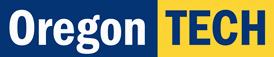Math 111 - College Algebra Gregg Waterman Department of Mathematics Oregon Institute of TechnologyChapters: Equations Solving equations, graphing solutions to equations in two unknowns. Introduction to Functions Working with functions, domain and range, behaviors of functions, solving problems with functions. Linear and Quadratic Functions Characteristics and applications of linear and quadratic functions. Polynomial and Rational Functions Polynomial and rational functions and their graphs, polynomial inequalities. More on Functions Combinations and transformations of functions, inverse functions. Exponential and Logarithmic Functions Characteristics and applications of exponential and logarithmic functions. Systems of Equations Systems of equations, matrix methods for systems of linear equations. Additional Resources: Syllabus Schedule This gives suggested or required activities for each class meeting. Final Exam Preparation Page Textbook The textbook for our course. Open in Firefox if you want to download the file to your computer. Here are the individual chapters, with the solutions for that chapter at the back of each: Desmos Online Grapher This is an online tool that allows you to plot graphs. Practice Exams: Winter 2013 Exams Exam 1 Part I and Part II Exam 2 Part I and Part II Exam 3 Part I and Part II Winter 2013 Exam Answers Return to Gregg's homepage